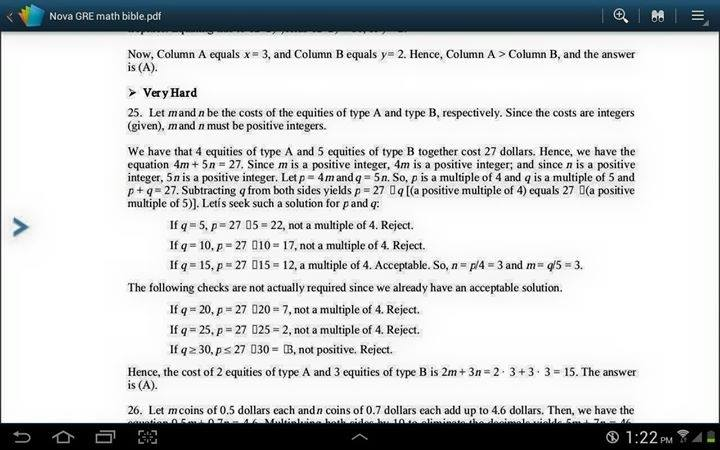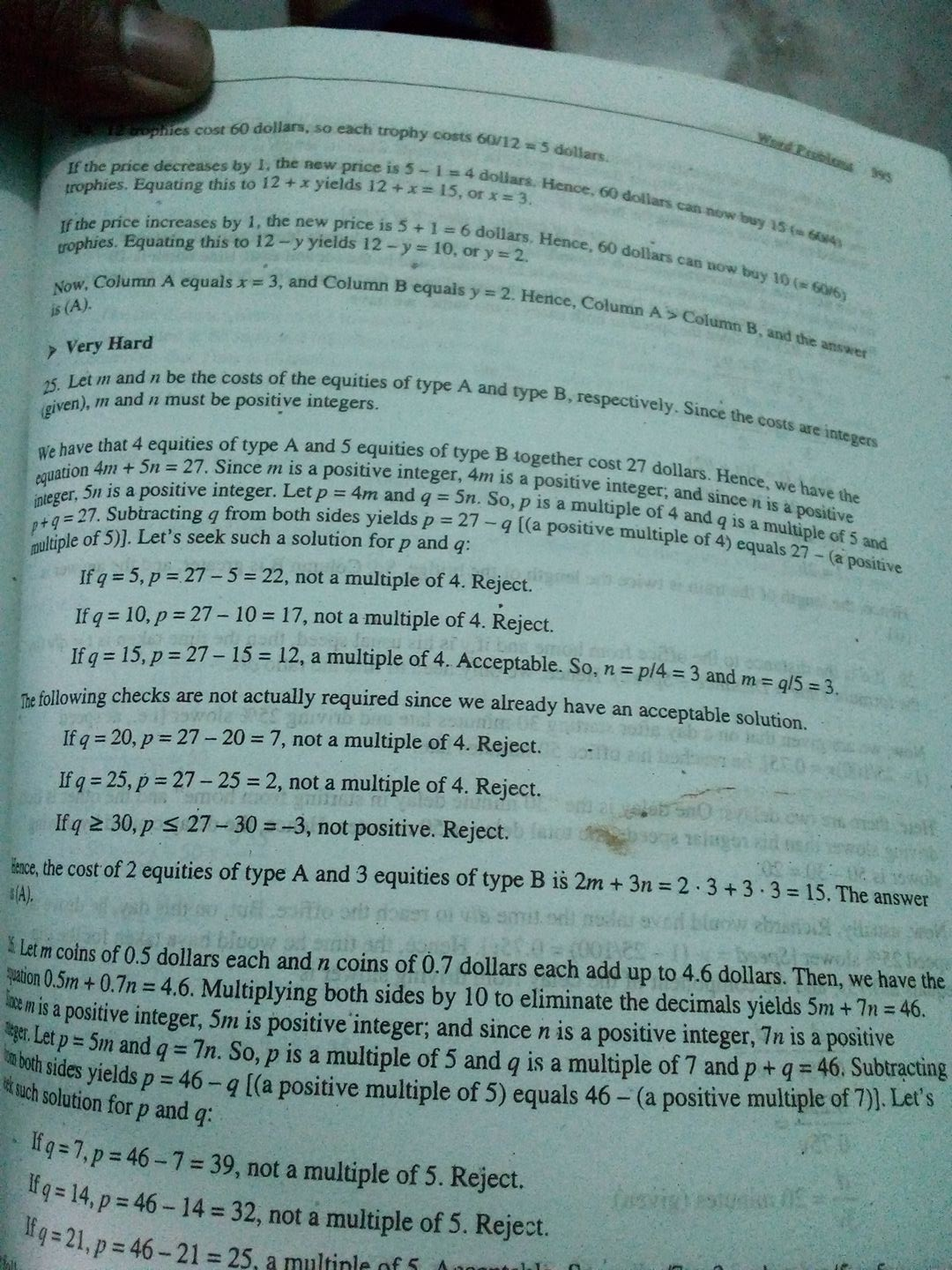Breaking News

# Islami Bank Written Exam Question Solution 2017

Bangladesh Islami Bank written Exam complete today . Now We published Islami Bank Written Exam Question Solution at ejobscircular.com . IBBL Math Question Solution also available now. Let`s check below this Full IBBL Job Question Solution on 07 April 2017.

## Islami Bank Written Exam Question SolutionMath:

The cost of A And B equities is the different of two positive integer.if sum of 4 equities of A and 5 equities of B is 27.what is the total cost of 2 equities of A and 3 equities of B?—-IBBL(PO)-07/04/2017

4A+5B=27
or, A=(27-5B)/4——–(i)
now, if B=1 then A=(27-5)/4=4.5 which is not integer
it is only possible when B=3 then A=3
so 2A+3B=2*3+3*3=15
Note: according to condition A&amp;B is different integer. but it is not possible to find out different value of A &amp; B

ibbl written puzzle solution..
1. I cannot seat
2. F and H must be seat
3. F and T cannot be selected
4. U is the 4 th one
5. 2 combination…

BBL (PO) Written GK Solution

1) Bengali Renessiance starts = Raja Rammohon Roy
2) Schedule Bank Regulated by = Bank Company Act 1991
3) Armed Forces Day = 21st November
4) @  = Domain Name
5) Monpura 70 = Zainul Abedin
6) 2019 Icc cricket world cup = England
7) Mongla port = Pashur River
8) VSAT = Very Small Aperture Terminal
9) IFC headquarters = Washington DC